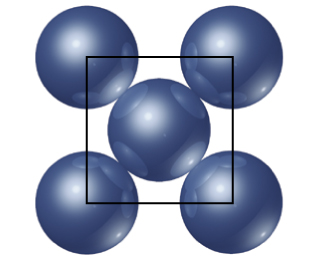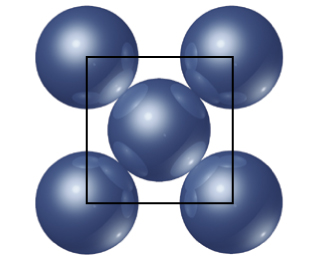# Problem: Iridium crystallizes in a face-centered cubic unit cell that has an edge length of 3.833 Å. The atom in the center of the face is in contact with the corner atoms, as shown in the drawing.Calculate the density of iridium metal.

###### FREE Expert Solution

We are being asked for the density of Iridium Ir, given the unit cell edge length of 3.833 Å.

Looking at the illustration, Iridium has a face-centered cubic structure.

Recall that the face-centered cubic lattice contains an atom in each of the faces of the cube and an atom in each of the corners

It looks like this:There are 4 atoms in a unit cell for a Face Centered Cubic structure (z=4).

An FCC unit cell contains four atomsone-eighth of an atom at each of the eight corners (8 x 1/8 = 1 atom from the corners) and one-half of an atom on each of the six faces (6 x 1/2 = 3 atoms from the faces).

95% (495 ratings)###### Problem Details

Iridium crystallizes in a face-centered cubic unit cell that has an edge length of 3.833 Å. The atom in the center of the face is in contact with the corner atoms, as shown in the drawing.

Calculate the density of iridium metal.Frequently Asked Questions

What scientific concept do you need to know in order to solve this problem?

Our tutors have indicated that to solve this problem you will need to apply the Unit Cell concept. You can view video lessons to learn Unit Cell. Or if you need more Unit Cell practice, you can also practice Unit Cell practice problems.

What professor is this problem relevant for?

Based on our data, we think this problem is relevant for Professor Halihan's class at OKSTATE.